# 前言

1. 首先，新闻推荐需要处理大量的文本信息，正与我未来方向(NLP)有较大关联
2. 新闻中包含着大量的实体，有利于探索基于知识(知识图谱)的推荐方法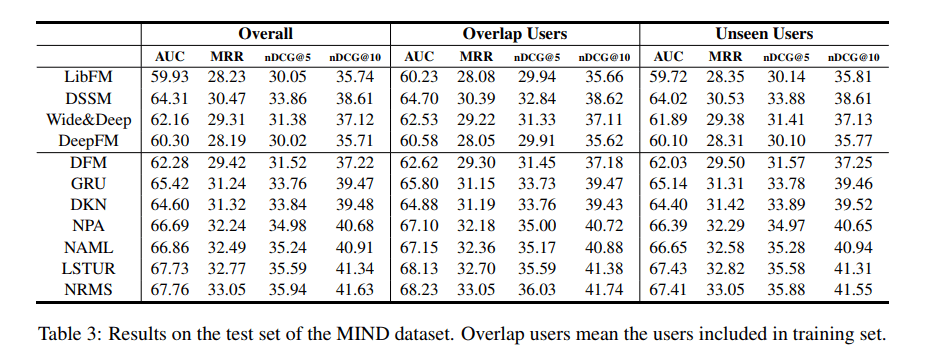# 0.几篇论文的共同点

1. 这四篇论文都是基于三个主要模块：新闻表示模型用户表示模型点击预测(包括之前的DKN也是)。其中，新闻表示模型通常都从新闻内容(如新闻标题，新闻类别，新闻内容，新闻内容中包含的实体)中学习，而用户表示通常从用户的浏览历史新闻中学习，点击预测即使用新闻表示和用户表示来计算用户点击这个新闻的概率
2. 这四篇论文都大量使用了Attention Mechanism注意力机制(DKN在对用户建模时也使用了)

# 1. NPA: Neural News Recommendation with Personalized Attention

## 1.1 核心思想

1. 一个新闻标题中的不同单词往往会对用户产生不同的影响
2. 并不是一个用户所浏览过的所有新闻都能反映他的偏好

## 1.2 模型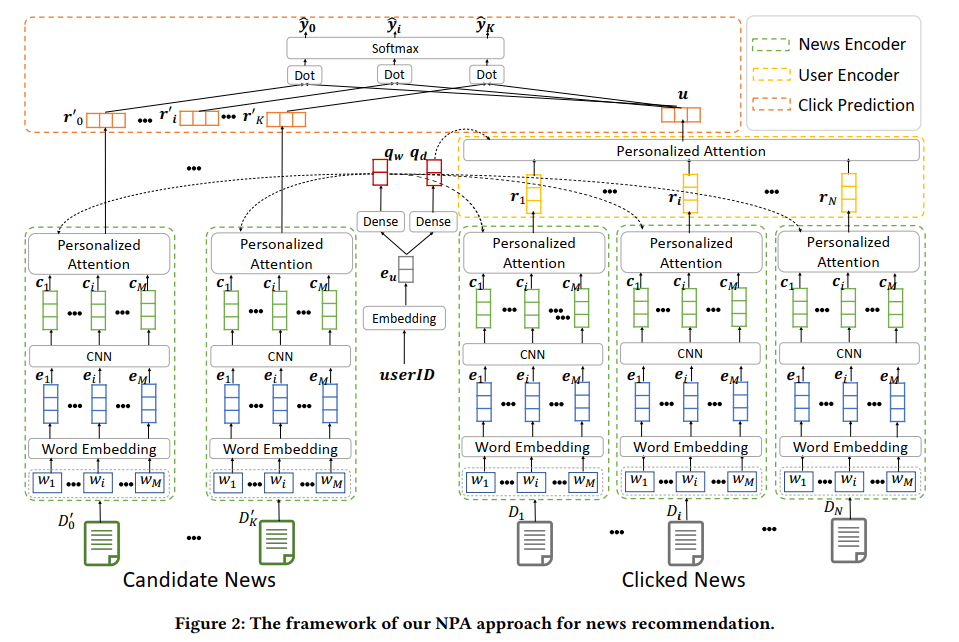### 1.2.1 新闻表示模型

• 词嵌入。即使用词向量(word2vec/glove)技术，将标题中每个单词映射成其对应的向量表示，这样，新闻标题就变成了一个词向量序列$E=(\bm{e_1},…,\bm{e_M})$
• 卷积神经网络。使用卷积神经网络的目的是想学习每个单词的局部上下文信息，也就是使用一个单词和其前后$k$个单词的表示，通过卷积操作来共同学习这个单词的表示。这样，得到一个新的单词表示序列$C=(\bm{c_1},…,\bm{c_M})$
• 单词级别的注意力机制，是本篇论文的核心之一。其目的是：为标题中的每个单词，通过注意力机制得到一个权重。这些权重就可以体现用户对每个单词的关注度，再将所有单词加权平均，得到新闻标题，也就是新闻的最终表示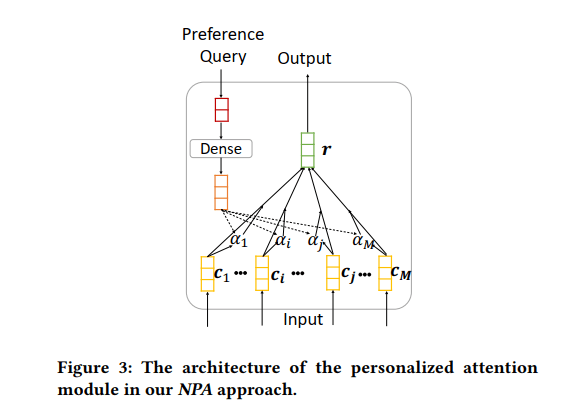1. 首先需要将每个用户的ID映射成一个向量$\bm{e_u}$(具体方法文中没有讲，可能是随机初始化最后学习得到的，需要去看代码了解)

2. 再将$\bm{e_u}$经过一个非线性映射，得到一个查询向量$\bm{q_w}=ReLU(\bm{V_w}\times\bm{e_u}+\bm{v_w})$，其中$\bm{V_w}$和$\bm{v_m}$都是模型参数(就是会不断被更新的辣种)，下面同理!

3. 最后使用查询向量$\bm{q_w}$先经过非线性映射，再与每个单词做点乘，最后使用$\mathrm{softmax}$函数归一化，得到每个单词的注意力。

\begin{aligned} &a_i=\bm{c_i}^\mathrm{T}tanh(\bm{W_p}\times\bm{q_w}+\bm{b_p})\\ &\alpha_i=\frac{exp(a_i)}{\sum_{j=1}^Mexp(a_j)} \end{aligned}
• 一个新闻最终的表示，就是所有单词的加权平均啦：$\bm{r_i}=\sum_{j=1}^M\alpha_j\bm{c_j}$

### 1.2.3 点击预测模型

\begin{aligned} &\hat y_i'=\bm{r_i'^\mathrm Tu}\\ &\hat y_i=\frac{exp(\hat y_i')}{\sum_{j=0}^Kexp(\hat y_j')}\\ &L=-\sum_{y_j\in S}log(\hat y_j) \end{aligned}

## 1.3 实验结果和其他有价值的东东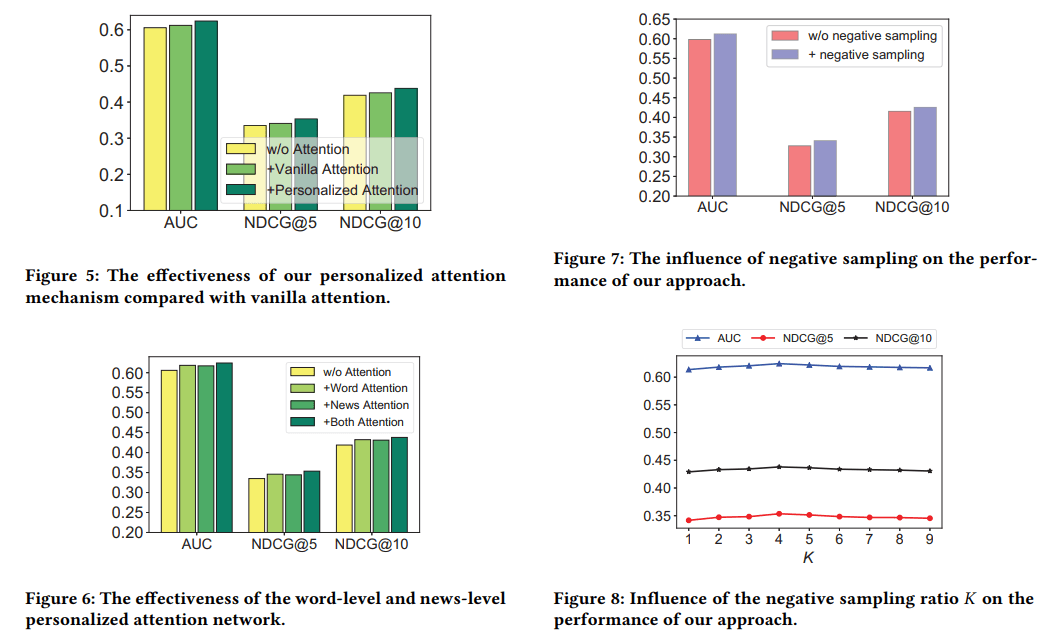# 2. NAML: Neural News Recommendation with Attentive Multi-View Learning

## 2.1 核心思想

1. 新闻包含多种成分，对学习其表示有所帮助(我理解为信息可以相互补充)
2. 新闻的不同成分通常会蕴含着不同特性(比如标题很简明扼要，内容会长而具体)

## 2.2 模型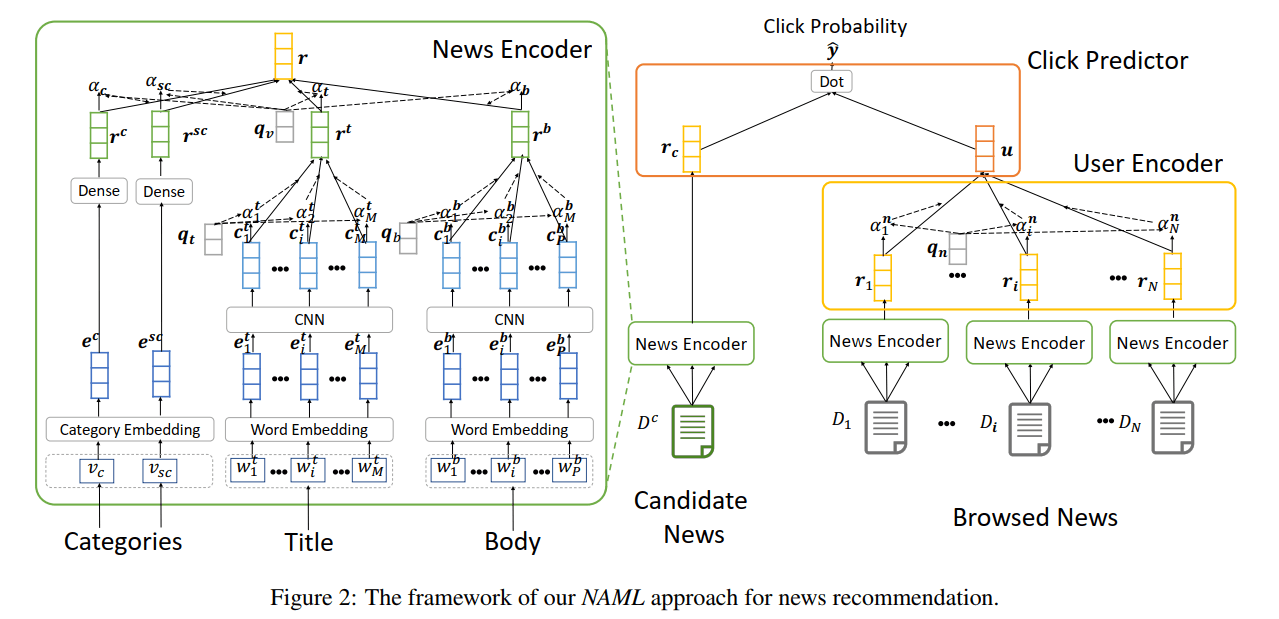### 2.2.1 新闻表示模型

1. 嵌入层: 将离散的ID转换为低维稠密向量$\bm{e^c}$和$\bm{e^{sc}}$
2. Dense层：将以上表示分别再经过非线性映射得到$\bm{r^c}$和$\bm{r^{sc}}$

## 2.3 实验结果和一些重要结论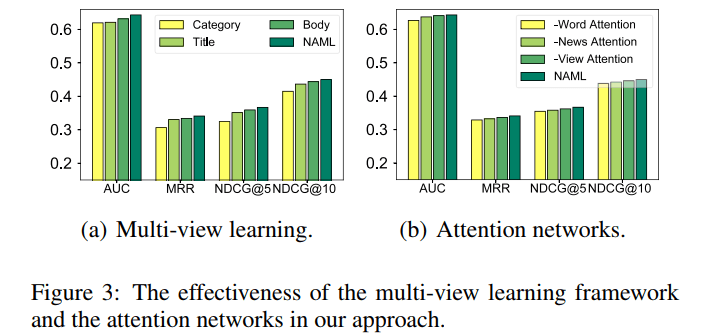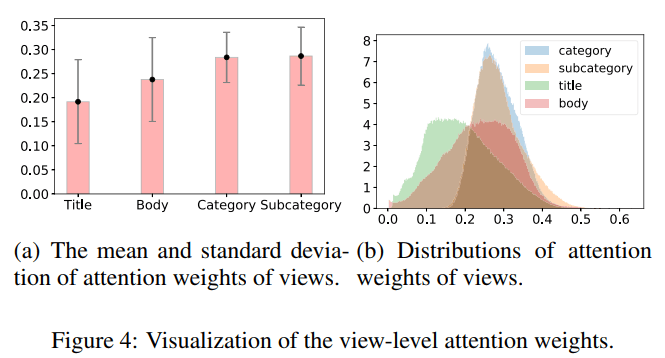# 3. LSTUR: Neural News Recommendation with Long- and Short-termUser Representations

## 3.2 模型

### 3.2.1 新闻表示模型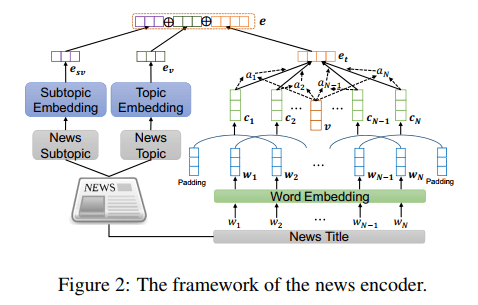### 3.2.2 用户表示模型

• 长期偏好表示： 作者使用用户ID的嵌入$\bm{u_l}$来作为用户的长期偏好。具体的步骤这里也讲了：随机初始化+在模型训练时自动学习得到(这也解释了之前一系列基于用户ID的表示的具体步骤)
• 短期兴趣表示： 作者使用了GRU(gated recurrent networks)来根据用户的浏览历史(按时间顺序)学习用户的短期兴趣表示。若新闻序列为$(\bm{e_1,e_2,…,e_k})$，有如下公式(即GRU的几个公式)：
\begin{aligned} &\bm{r_t}=\sigma(\bm{W_r[\bm{h_{t-1},e_t}]}),\\ &\bm{z_t}=\sigma(\bm{W_z[\bm{h_{t-1},e_t}]}),\\ &{\bm{\tilde h_t}}=tanh(\bm{W_{\tilde{h}}[r_t\odot h_{t-1},e_t]}),\\ &\bm{h_t}=\bm{z_t}\odot\bm{h_t}+(\bm{1-z_t})\odot\bm{\tilde{h_t}}\\ &\bm{u_s=h_k} \end{aligned}
• 长期表示与短期表示结合： 作者提出了两种方法，一种是使用用户的长期表示来初始化GRU的初始状态，取GRU的最终状态来作为用户表示(LSTUR-ini)，另外一种是直接将用户的长期表示和GRU的最终状态拼接得到用户表示(LSTUR-con)。在实验中，两种方法的得分基本一致，但con方法更稳定。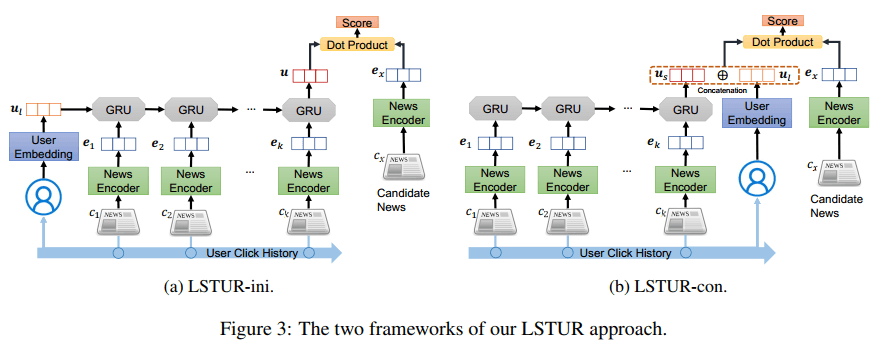# 4. NRMS: Neural News Recommendation with Multi-Head Self-Attention

## 4.2 模型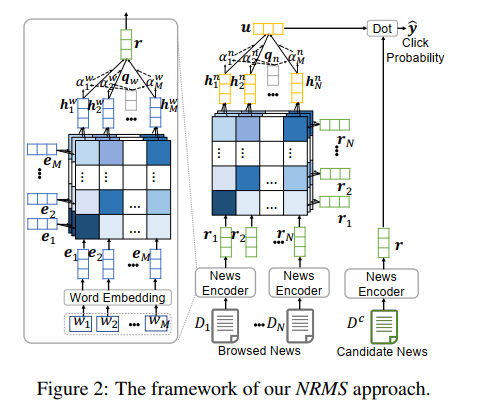### 4.2.1 多头自注意力

\begin{aligned} &\alpha_{i,j}^k=\frac{\mathrm{exp}(\bm{e_i^\mathrm{T}}\bm{Q_k^we_j})}{\sum_{m=1}^M\mathrm{exp}(\bm{e_i^\mathrm{T}}\bm{Q_k^we_m})}\\ &\bm{h_{i,j}^w}=\bm{V_k^w}(\sum_{j=1}^M\alpha_{i,j}^k\bm{e_j})\\ &\bm{h_i^w}=[\bm{h_{i,1}^w;h_{i,2}^w;...;h_{i,h}^w}] \end{aligned}

## 4.3 改进思路

• 在使用self-attention的同时考虑单词或新闻的位置信息，即引入位置编码
• 考虑使用新闻的多种成分，但如果引入像新闻内容这样的长序列，这是对自注意力机制的一种挑战